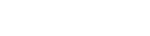﻿ 贴片电阻值/代码计算器 - ASCII码

#### 电阻值：

3位代码

3 位代码示例：
220 = 22 x 100 (100=1) = 22Ω（不是 220Ω 呵）
471 = 47 x 101 (101=10) = 470Ω
102 = 10 x 102 (102=100) = 1000Ω 或 1kΩ
3R3 = 3.3Ω

4位代码
4 位代码用于标记精密电阻。 和上面的计算方法类似，唯一的区别是有效位数：前三个数字告诉我们有效数字，第四个是乘数，表示三个有效数字必须乘以的十的幂 （或要添加多少个零）。 小于 100 欧姆的电阻用字母“R”标记，表示小数点的位置。
4 位代码示例：
4700 = 470 x 100 (100=1) = 470Ω（不是 4700Ω 呵）
2001 = 200 x 101 (101=10) = 2000Ω 或 2kΩ
1002 = 100 x 102 (102=100) = 10000Ω 或 10kΩ
15R0 = 15.0Ω

1 (MΩ) = 1000 (kΩ)
1 (kΩ) = 1000 (Ω)